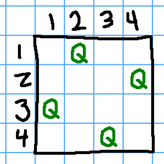# Assignment 3

Due Wednesday 3 October at 1am

Open any file within your `cs162` folder in the editor, and then choose the “Sync with git” option from the Tools » External Tools menu.

Now you should have an `a3` folder, with three sub-folders: `lsat1`, `lsat2`, and `queens`. These are solutions to problems that use the AC3 (arc consistency) algorithm.

# Queens

Open `queens/queens.cpp` and run it. You should see output that ends like this:

``````  Satisfiable:
1: { 2 }
2: { 4 }
3: { 1 }
4: { 3 }``````

This corresponds to the positions of queens on a 4x4 chess board: the queen in row 1 is in column 2, the queen in row 2 is in column 4, etc. This solution corresponds to the following diagram, but there are other valid solutions too.# Puzzle

We can use the same technique to solve other sorts of constraint problems. Here’s a typical logic puzzle from the LSAT or GRE exam:

An advertising executive must schedule the advertising during a particular television show. Seven different consecutive time slots are available for advertisements during a commercial break, and are numbered one through seven in the order that they will be aired. Seven different advertisements – A, B, C, D, E, F, and G – must be aired during the show. Only one advertisement can occupy each time slot. The assignment of the advertisements to the slots is subject to the following restrictions:

1. A and C must occupy consecutive time slots.

2. A must be aired during an earlier time slot than G.

3. C must be aired during a later time slot than E.

4. If E does not occupy the fourth time slot, then D must occupy the fourth time slot.

5. G and F cannot occupy consecutively numbered time slots.

You can find an implementation of this puzzle in `lsat1/lsat1.cpp`. The constructor, `lsat_schedule:: lsat_schedule` (around line 43) sets up the domains and the interference graph. There are no unary constraints, so the initial domains are these:

``````  A: { 1 2 3 4 5 6 7 }
B: { 1 2 3 4 5 6 7 }
C: { 1 2 3 4 5 6 7 }
D: { 1 2 3 4 5 6 7 }
E: { 1 2 3 4 5 6 7 }
F: { 1 2 3 4 5 6 7 }
G: { 1 2 3 4 5 6 7 }``````

Because the slots into which each ad is scheduled must all be distinct, the interference graph is fully connected. (Choosing slot 4 for advertisement B eliminates slot 4 from the domains of all other advertisements.)

Here is the implementation of the rules in the `last_schedule:: check` method. This method takes two key-value pairs. It should return `false` if the key-value pairs have any settings that are incompatible with the rules.

``````bool lsat_schedule::check(Key k1, Val v1, Key k2, Val v2) const
{
// All must be distinct
if(v1 == v2) return false;

// (1) A and C must occupy consecutive time slots.
if((k1 == 'A' && k2 == 'C') || (k1 == 'C' && k2 == 'A')) {
if(abs(v1-v2) != 1) return false;
}
// (2) A must be aired during an earlier time slot than G.
if(k1 == 'A' && k2 == 'G') {
if(v1 >= v2) return false;
}
if(k1 == 'G' && k2 == 'A') {
if(v2 >= v1) return false;
}
// (3) C must be aired during a later time slot than E.
if(k1 == 'C' && k2 == 'E') {
if(v1 <= v2) return false;
}
if(k1 == 'E' && k2 == 'C') {
if(v2 <= v1) return false;
}
// (4) If E does not occupy the fourth time slot, then D must occupy the
// fourth time slot.
if((k1 == 'E' && k2 == 'D') || (k1 == 'D' && k2 == 'E')) {
if(v1 != 4 && v2 != 4) return false;
}
// (5) G and F cannot occupy consecutively numbered time slots.
if((k1 == 'G' && k2 == 'F') || (k1 == 'F' && k2 == 'G')) {
if(abs(v1-v2) < 2) return false;
}
return true;
}``````

With that implementation of the rules, the AC3 algorithm easily finds the following solution:

``````  Satisfiable:
A: { 2 }
B: { 6 }
C: { 3 }
D: { 4 }
E: { 1 }
F: { 5 }
G: { 7 }``````

Your task is to solve a second LSAT-style puzzle using the AC3 algorithm. It is partially set up for you in `lsat2/lsat2.cpp`. You just have to finish the `::check` method. The puzzle is this:

A veterinarian employed by the city zoo feeds the same animals every morning. The animals — falcons, gorillas, hyenas, iguanas, jaguars, kangaroos, lemurs, and monkeys — are fed one at a time and exactly once every morning. To ensure the animals are fed in an acceptable order, the following requirements must be satisfied:

1. The gorillas are fed second or sixth. — Unary Constraint

2. Exactly two animal groups must be fed between the feedings of the hyenas and the gorillas.

3. The falcons must be fed immediately before or immediately after the gorillas.

4. The monkeys are fed earlier than the gorillas.

5. The monkeys are fed after the kangaroos.